# How to Use Gross Sales Formula in Excel

This guide will discuss how to use the gross sales formula in Excel to calculate gross sales.

When we need to calculate the gross sales to keep track of a company’s financial situation, we can easily do it using different gross sales formulas in Excel.

Gross sale is a really common concept in the world of finance. It is a great way to monitor the financial health of a company. However, it can be difficult to calculate it, especially for those who are not familiar with the concept.

While Excel does not have a  built-in formula for calculating gross sales,  we can make use of existing functions to easily perform the formula and get the gross sales in different situations. In this case, we will utilize the `SUM` function in Excel.

In this guide, we will provide a step-by-step tutorial on how to use the gross sales formulas in Excel. Additionally, we will explore the syntax and a real example of using the gross sales formula.

Great! Let’s dive right in.

## The Anatomy of the SUM Function

The syntax or the way we write the `SUM` function is as follows:

`=SUM(number1, [number2])`
• = the equal sign is how we start any function in Excel.
• number1 is a required argument. This refers to 1 to 255 numbers we want to sum. Furthermore, logical values and text are ignored in cells, including if-typed as arguments.
• number2 is an optional argument. This acts as a supplementary argument. It also refers to another 1 to 255 numbers we want to sum. Similarly, logical values and text are ignored in cells, including if-typed as arguments.

## The Gross Sales Formula

Gross sales represent the total revenue a business generates from the sale of its goods or services before deducting expenses, taxes, or discounts.

The most common gross sales formula is:

`gross sales = net sales+sales returns+discounts+allowances`

Now let’s take turns understanding each term in the formula.

Net sales refer to the total revenue a company earns from the sale of its goods or services after subtracting any deductions such as discounts, returns, or allowances. It represents the actual amount of money received by the company from its customers.

The net sales formula is as follows:

`net sales = gross sales-sales return-discounts-allowances`

Sales returns are the products that customers have returned to the company for several reasons. When a customer returns a product, we must deduct the sales revenue from the amount of the returned product.

Discounts refer to reductions in the price of a product or service given to customers to increase purchases. On the other hand, allowances are reductions in the price of a product or service given by the company as compensation.

## A Real Example of Using Gross Sales Formula in Excel

There are different situations in calculating gross sales in Excel. Let’s say we want to calculate total gross sales by adding the daily gross sales. Our initial data set would look like this:In this example, we will utilize the formula:

`total gross sales = sum of all daily gross sales`. In the sheets, our formula would be `=SUM(C2:C6)`

We will use the `SUM` function to easily sum up all the daily gross sales. Our final data set would look like this: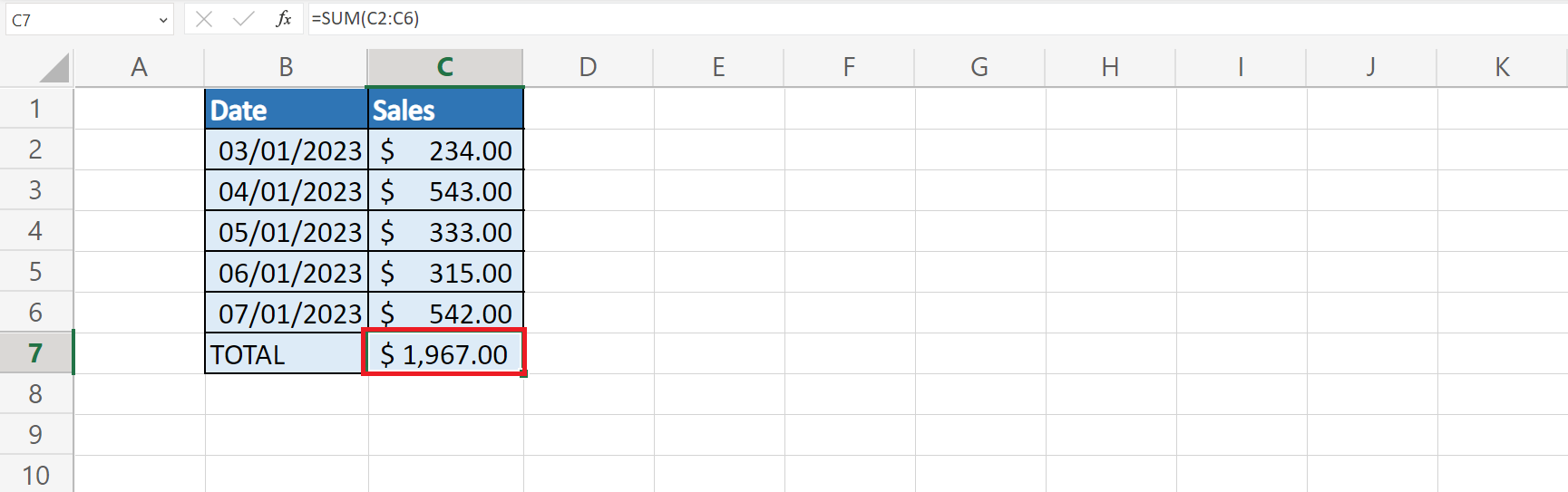Another situation would be calculating the gross sales with discounts, sales returns, or allowances on sales. Our initial data set would look like this:For this instance, we will use the formula:

`gross sales = net sales+discounts/returns/allowances`. In our sheet, the formula would be `=C10+D10 `and `=SUM(E10:E12)`.

Afterward, we will use the same total gross sales formula. This would give us an output like the one below.Lastly, we can also calculate gross sales with percentage discounts on sales. Our initial data set would look like this:In this case, we will use the formula:

`gross sales = net sales*(100/100-discount)`. The formula in the sheet would be `=C15*(100/(100-15))` and `=SUM(E15:E17)`.

Then, we will use the total gross sales formula to get the final result. Our final data set would look like this: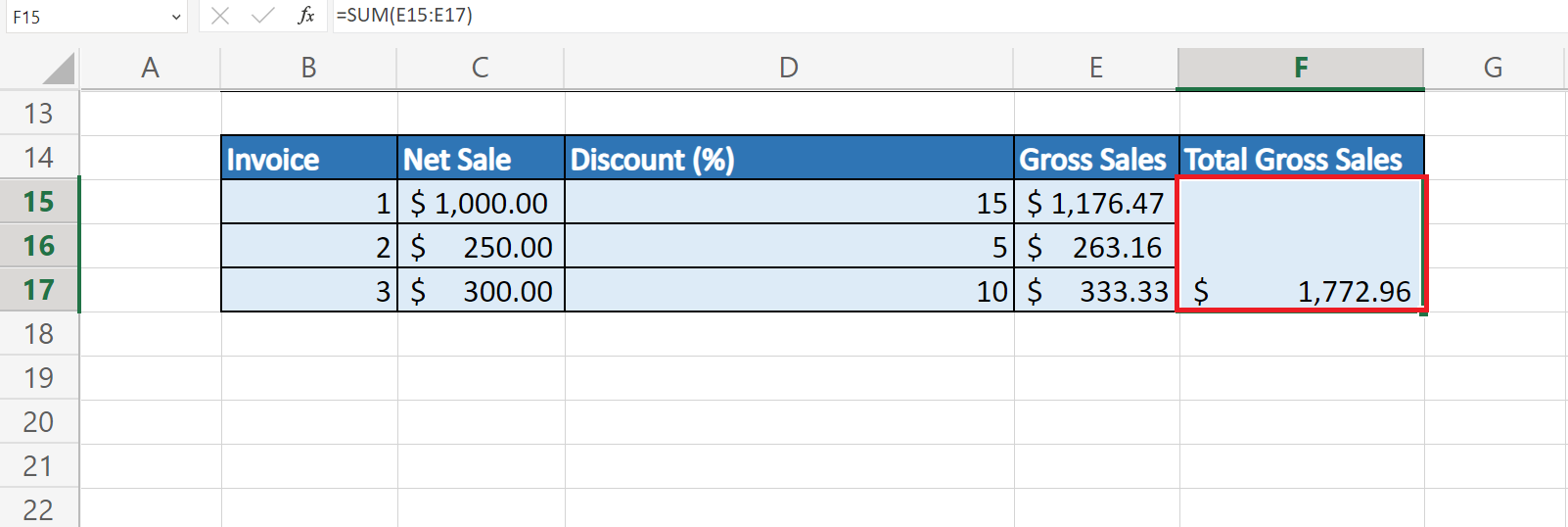Amazing! Now we can dive into the steps of using the gross sales formula in Excel.

## How to Use Gross Sales Formula in Excel

1. First, we will calculate the total gross sales. We will use the formula total gross sales = sum of all daily gross sales. To do this, we will type in an equal sign and the `SUM` function to get “=SUM(“.2. We will select all the daily gross sales in the table. Then, we will get “=SUM(C2:C6)”.3. Lastly, we will press the Enter key to get the result.4. Next, we will calculate gross sales with discounts, sales returns, or allowances. Firstly, we will select the net sale and add the discount. This will give us the formula “=C10+D10”. Then, press the Enter key to get the sum.5. Drag down the Fill Handle tool to apply the same formula to the rest of the cells.6. Then, we will sum all the individual gross sales to get the total sales. We will type in the formula “=SUM(E10:E12)”.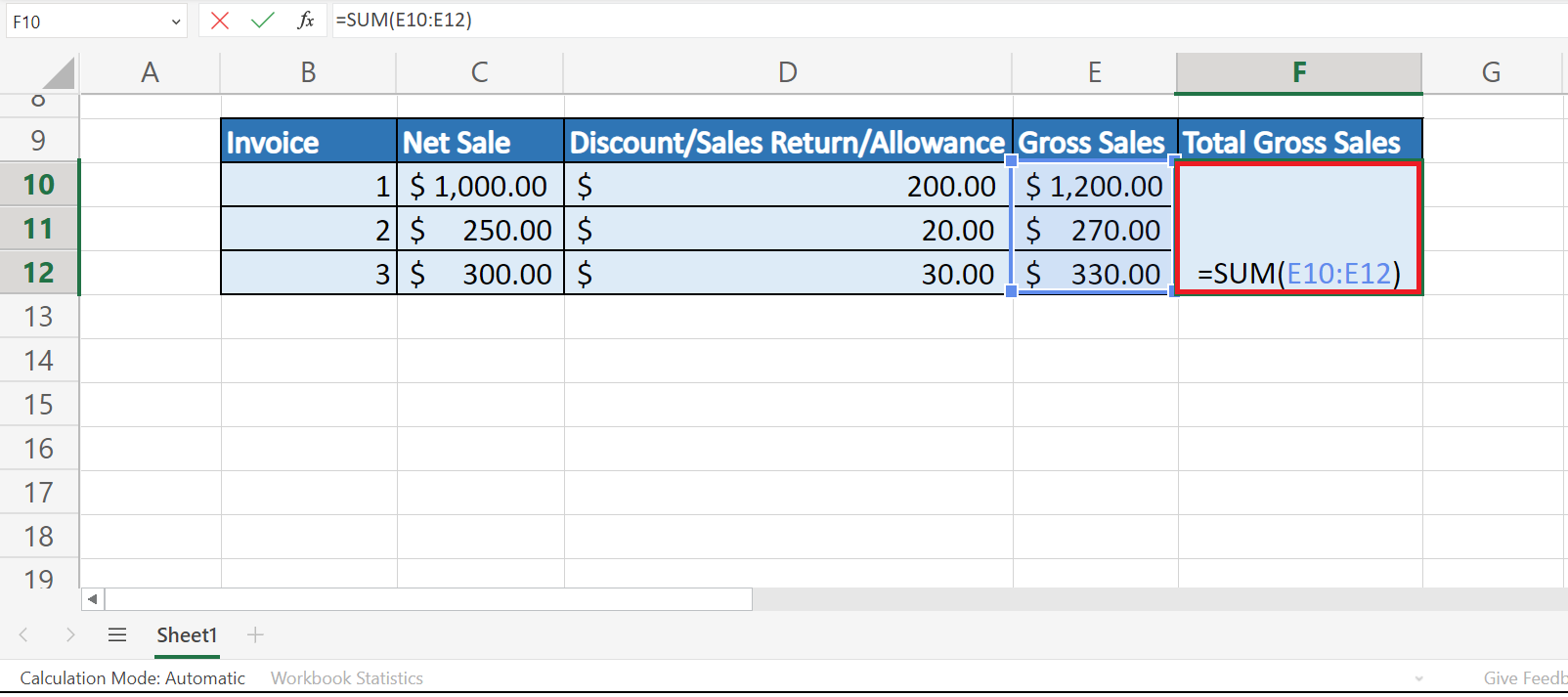7. We will press the Enter key to return the results.8. We will calculate the gross sales with percentage discounts on sales. We will use the formula gross sales = net sales* (100/100-discount). In the sheet, select the net sale value and the discount percentage value to get this formula “=C15*(100/(100-D15))”.9. Drag down the Fill Handle tool to copy the formula to the other cells.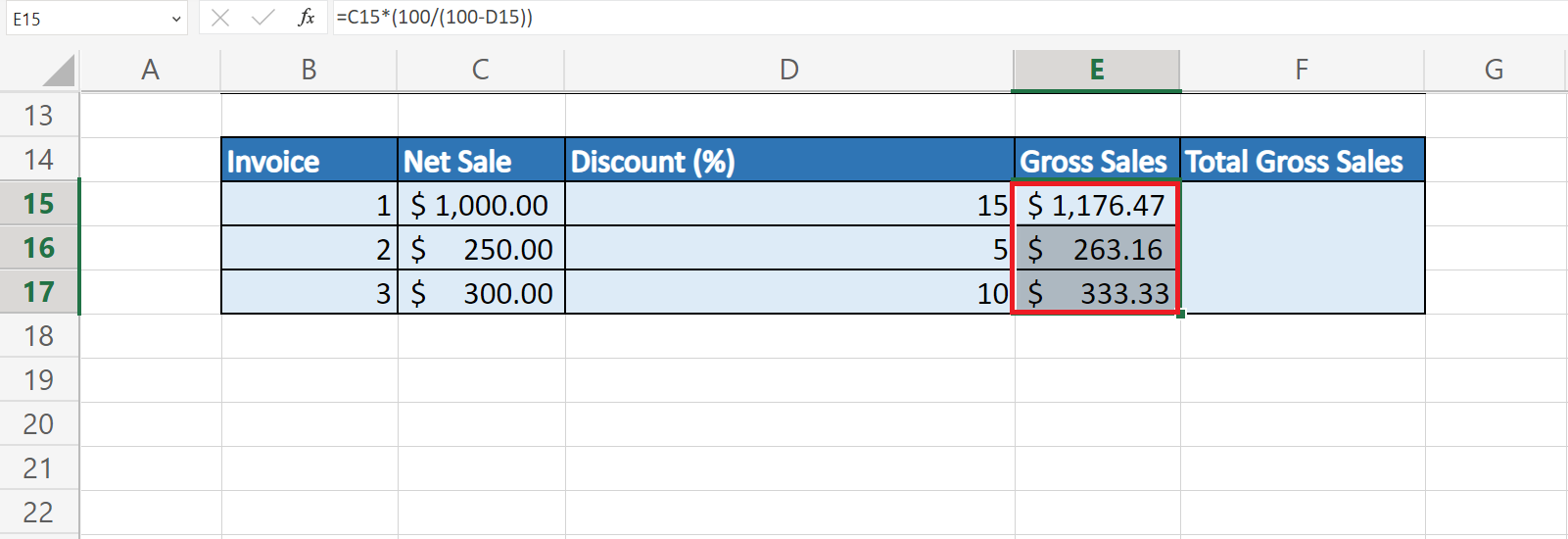10. Then, we will sum up all the values. We will type in the formula “=SUM(E15:E17)”.11. We will press the Enter key to return the output.And tada! We have successfully used the gross sales formula in Excel.

You can apply this guide whenever you need to calculate the gross sales for your company’s finance. You can now use the `SUM` function and the various other Microsoft Excel formulas available to create great worksheets that work for you.

## FAQs:

1. How to calculate gross sales by including prepayment discounts?

You need to first determine the total sales amount before any discounts are applied. Then, you calculate the prepayment discount, which can be a fixed amount or a percentage. This discount is subtracted from the total sales amount to arrive at the gross sales figure.

That’s pretty much it! Make sure to subscribe to our newsletter to be the first to know about the latest guides and tutorials from us.Our goal this year is to create lots of rich, bite-sized tutorials for Google Sheets users like you. If you liked this one, you'll love what we are working on! Readers receive early access to new content.

##### You May Also Like## How to Multiply Column by a Constant in Excel

This guide will discuss how to multiply a column by a constant in Excel using three easy and…## How to Calculate the Mean Absolute Deviation in Excel

This guide will explain how to calculate the mean absolute deviation in Excel. Excel is a popular tool…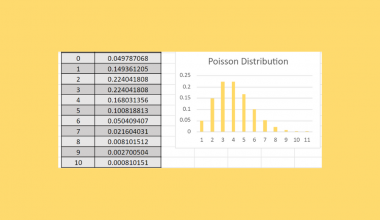## How to Plot Poisson Distribution in Excel

This guide will explain how to plot Poisson distribution in Excel using the POISSON.DIST function. Essentially, we first…## How to Do Conditional Formatting for Blank Cells in Excel

This guide will explain how to do conditional formatting for blank cells in Excel using two simple methods.…